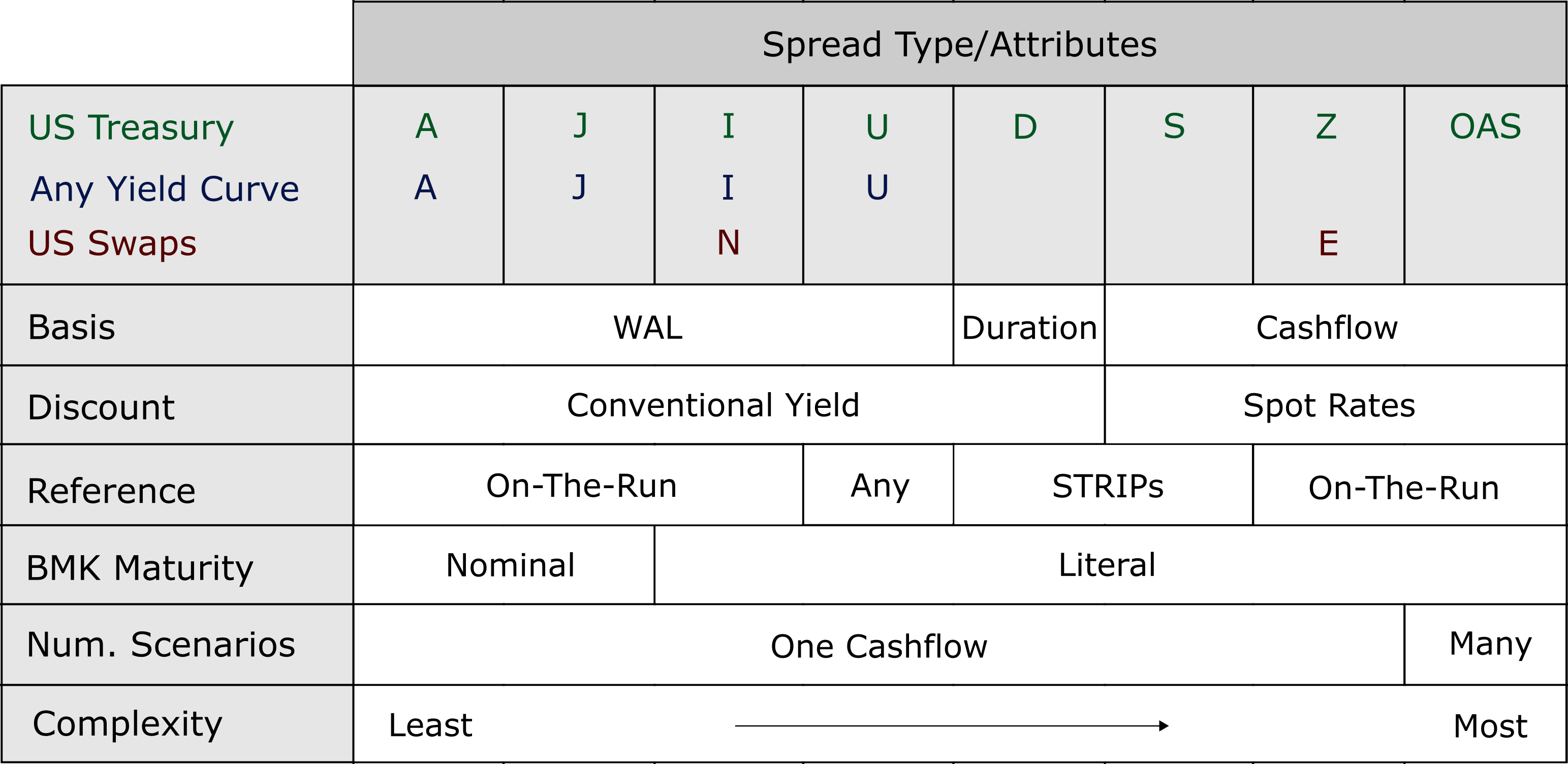I: Conventional yield spread to Interpolated Yield Curve

J: Conventional yield spread to Interpolated Nominal Yield Curve

A: Conventional yield spread to nominal (Actual) Treasury benchmarks

U: Conventional yield spread to specific (Unique) benchmark

Z: Cashflow spread to implied spot curve

S: Cashflow spread to actual US STRIP Curve

D: Conventional yield spread to Duration-matched US STRIP benchmark

N: Conventional yield spread to swap curve

E: Cashflow spread to Eurodollar spot curve (IMM)

The primary distinction of these methods is whether spread is with respect to yield or cashflow. A yield spread compares on yield to another, with both computed in a conventional manner. However, a cashflow spread discounts each component cashflow at its own spot rate. The most popular spread choices are:

US Treasury, Coupon curve Yield Spread: I

US Treasury, Spot rates Cashflow Spread: Z

US Swaps, Coupon curve Yield Spread: N

US Swaps, Spot rates Cashflow Spread: EA-spread: Conventional yield spread to a specific on-the-run Treasury benchmark, such as 5-year or 10-year. Any benchmark can be specified, including a hypothetical maturity - in which case the associated yield is interpolated based upon nominal benchmark maturities.

J-spread: A variation of A-spread wherein a hypothetical (interpolated) maturity point is automatically set to the mortgage's WAL.

I-spread: Similar to J-spread, except that it anchors off the literal maturity of the benchmarks - rather than their nominal values.